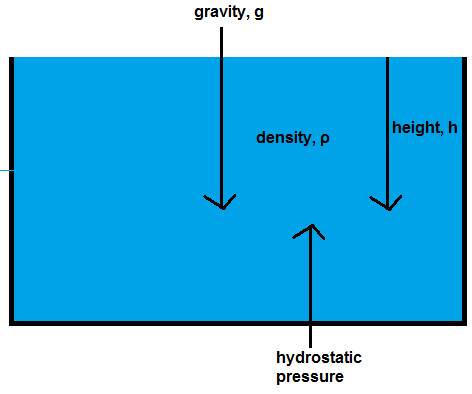﻿ Hydrostatic Pressure Calculator

# Hydrostatic Pressure CalculatorEnter the height, h: m Enter the pressure, ρ: kg/m3 Enter the gravity, g: m/s2

0.00 N/m2 or Pa

This hydrostatic pressure calculator calculates the hydrostatic pressure of a fluid at equilibrium.

The hydrostatic pressure is the pressure at a point that a fluid at rest exerts due to the force of gravity.

The hydrostatic pressure is affected by 3 factors, the earth's gravitational pull, g, the height at which the fluid is from the surface, h, and the density, ρ of the fluid, according to the formula, p= hρg.

The earth's gravitational force is a downward force. It pushes the fluid downward with a pull of 9.81m/s2. The density of the fluid will also affect how much pressure is weight down on it. The more dense a fluid is, the more pressure that is exerted on it. The less dense, the less pressure. The last factor affecting how much hydrostatic pressure a fluid exerts is the height it is from the surface. The greater the height the fluid is from the surface, the more fluid from above is pushing it down. So the greater the height, the greater the pressure. Thus, hydrostatic pressure and height have a direct relationship, as the other 2 variables do.

To use this calculator, a user just enters the height, h, the density, ρ, and the gravitational force, and click the 'Calculate' button, and the resultant value, hydrostatic pressure will be computed and shown. The unit of hydrostatic pressure is N/m2 or pascals.

Hydrostatic pressure can be important with electronics that need to be submerged in water, such as waterproof devices. Since the fluid is at equilibrium, the hydrostatic pressure is equal to the amount of pressure that is being applied to the object. This can be important when pressure is a factor in device function.

Example Calculation

If a fluid at rest is 10m from the surface of a fluid with a density of 1000kg/m3, what is its hydrostatic pressure?

So based on the values of the parameters, we use the equation p= hρg and substitute in the values into the equation.

p= (10m)(1000kg/m3)(9.81m/s2)= 98,100 N/m2 or Pa